• keywords：Word, Pseudocode 三种方式： 1.latex 需要安装，宏包导入不对或者冲突的话，会导致莫名其妙的报错，debug的时候一头雾水。 2.Aurora 易用性介于两者之间，可直接作为插件在word文档编译，推荐使用。 3....

keywords：Word, Pseudocode

三种方式：
1.latex 需要安装，宏包导入不对或者冲突的话，会导致莫名其妙的报错，debug的时候一头雾水。
2.Aurora 易用性介于两者之间，可直接作为插件在word文档编译，推荐使用。
3.overleaf 在线编译最方便
附上链接：https://cn.overleaf.com/

注1：其中前两个可插入到word文档（可编辑），使用overleaf只能生成pdf，自行转换吧。哪位同学知道怎么用overleaf插入到word请赐教，毕竟在线编辑还是最方便的嘛（懒汉的心声哈哈）
注2：前些年的网上代码大多不可用，大家不用费劲调试了。
挖个坑
争取月底填

终于准备写一些东西了，不准备做技术向博主，想到什么写什么吧，权当记录生活。像工程开发、职业生活、异构无线网络垂直切换方向的研究探讨、健身、打球、旅行、情感、杂记等等吧。争取至少周更吧。

第一次写就不讲究排版了，希望能陪我见证自己的成长。

博客消息可能不能及时看到，留个微信吧：cqu1995

2020/10/11

展开全文ieee论文
• Office之word中插入Latex伪代码

万次阅读 热门讨论 2019-01-24 18:34:49
但有时我们必须要使用word，那么怎么在word里插入Latex的伪代码呢？ 下载Aurora.Aurora是一个很好用的公式和伪代码编辑器。 安装后打开word，如果有插件直接在插件里打开，没有的话就在插入-对象-Aurora ...

latex一直是一个高冷的排版工具，写出的伪代码可读性强，样式美观。
但有时我们必须要使用word，那么怎么在word里插入Latex的伪代码呢？

1. 下载Aurora.Aurora是一个很好用的公式和伪代码编辑器。自行搜索下载~
2. 安装后打开word，如果有插件直接在插件里打开，没有的话就在插入-对象-Aurora equation打开
3. 导入包：Properties → \rightarrow packages粘贴一下的包：
\usepackage{amsmath}
\usepackage{amssymb}
% \usepackage{euler}
\usepackage{amsfonts}
\usepackage{mathrsfs}

\renewcommand{\algorithmicrequire}{\textbf{Input:}}
\renewcommand{\algorithmicensure}{\textbf{Output:}}

\usepackage{multirow}
\usepackage{CJK}
\usepackage{algorithm}
\usepackage{algorithmic}

\providecommand{\abs}{\left\lvert#1\right\rvert}
\providecommand{\norm}{\left\lVert#1\right\rVert}

1. 正文可以复制代码（以下为参考示例）
\renewcommand{\thealgorithm}{1}   %定义算法的编号
\begin{algorithm}[H]
\caption{Doppler Profile Extraction}
\begin{algorithmic}
\REQUIRE{Spectrogram matrix $P$, centre frequency bin $cf$}
\ENSURE{Doppler shift profile $DopShift$}\\
\STATE{colNum=getColumNum($P$);} \\
\STATE{$DopShift$(1:colNum)=$cf$;}  //initialization \\
\FOR{i=1:colNum}
\STATE row=getNonNullRows(col(i)); // get non-null rows of the $i$th col\\
\IF{isNotEmpty(row)}
\STATE{meanValue=mean(row);}\\
\ELSE
\STATE{meanValue\textgreater cf}\\
\STATE{$DopShift$(i)=max(row);}\\
\STATE{$DopShift$(i)=min(row);}\\
\ENDIF
\ENDFOR
\STATE{$DopShift$ = SMA($DopShift$);} // SMA represents smoothed moving average filter\\
\STATE{Return $DopShift$;}
\end{algorithmic}
\end{algorithm}

几点说明：

• Aurora的伪代码按照的是\end{algorithmic}包语法，不是\end{algorithm2e}。如果使用2e包写的latex，需要再改一下。具体不同请看上述代码示例或者查看{algorithmic}包介绍, 或者这篇.
• 命令要大写，如STATE;
• 如果出现问题，请仔细检查自己的代码是否和上述示例一致。

得到结果预览如下：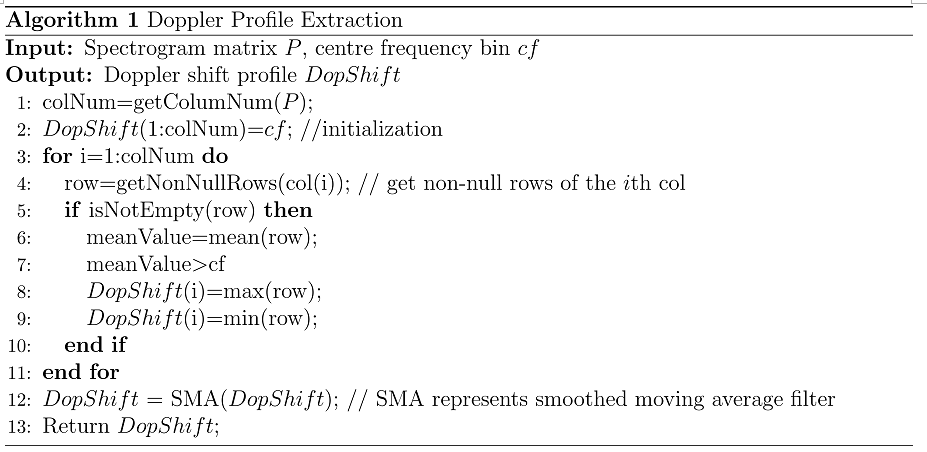展开全文latex matlab
• 在word中插入latex伪代码

千次阅读 2020-03-22 20:25:59
使用word时，需要插入latex样式的伪代码，如下所示： 安装后打开word，如果有插件直接在插件里打开，没有的话就在插入-对象-Aurora equation打开。 导入包：Properties→packages粘贴一下的包： \usepackage{...

使用word时，需要插入latex样式的伪代码，如下所示：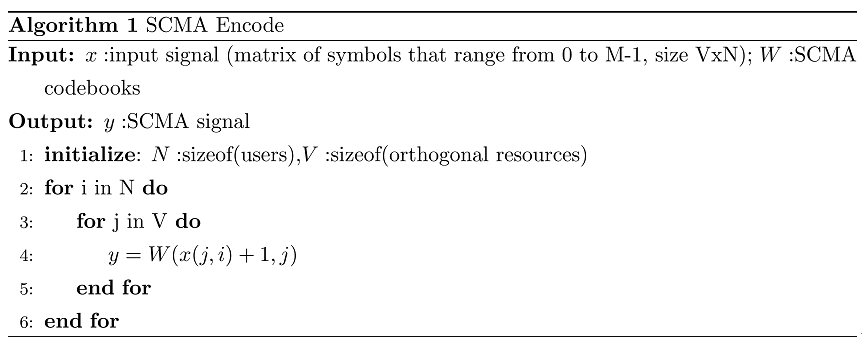安装后打开word，如果有插件直接在插件里打开，没有的话就在插入-对象-Aurora equation打开。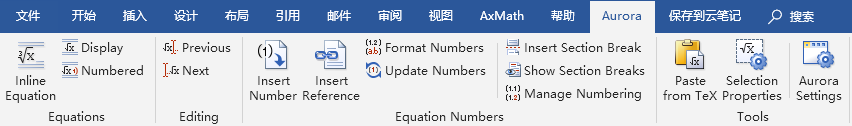导入包：Properties→packages粘贴一下的包：

\usepackage{amsmath}
\usepackage{amssymb}
% \usepackage{euler}
\usepackage{amsfonts}
\usepackage{mathrsfs}

\renewcommand{\algorithmicrequire}{\textbf{Input:}}
\renewcommand{\algorithmicensure}{\textbf{Output:}}

\usepackage{multirow}
\usepackage{CJK}
\usepackage{algorithm}
\usepackage{algorithmic}

\providecommand{\abs}{\left\lvert#1\right\rvert}
\providecommand{\norm}{\left\lVert#1\right\rVert}

正文可以复制代码（以下为参考示例）

\renewcommand{\thealgorithm}{1}   %定义算法的编号
\begin{algorithm}[H]
\caption{Doppler Profile Extraction}
\begin{algorithmic}
\REQUIRE{Spectrogram matrix $P$, centre frequency bin $cf$}
\ENSURE{Doppler shift profile $DopShift$}\\
\STATE{colNum=getColumNum($P$);} \\
\STATE{$DopShift$(1:colNum)=$cf$;}  //initialization \\
\FOR{i=1:colNum}
\STATE row=getNonNullRows(col(i)); // get non-null rows of the $i$th col\\
\IF{isNotEmpty(row)}
\STATE{meanValue=mean(row);}\\
\ElSE
\STATE{meanValue\textgreater cf}\\
\STATE{$DopShift$(i)=max(row);}\\
\STATE{$DopShift$(i)=min(row);}\\
\ENDIF
\ENDFOR
\STATE{$DopShift$ = SMA($DopShift$);} // SMA represents smoothed moving average filter\\
\STATE{Return $DopShift$;}
\end{algorithmic}
\end{algorithm}

展开全文• 最近论文中要插入伪代码，网上主流的方法都是Aurora，但自己安装的时候遇到各种问题。。。这个古老的插件实在是太麻烦了。 正常安装的话应该是只支持32位的Office（这年头了谁还用32位啊）。 操作系统：Win10 ...

最近论文中要插入伪代码，网上主流的方法都是Aurora，但自己安装的时候遇到各种问题。。。这个古老的插件实在是太麻烦了。
正常安装的话应该是只支持32位的Office（这年头了谁还用32位啊）。

操作系统：Win10
Office版本：2019, 64位

需要安装Aurora+MikTex2.9，下载地址

1 安装

1. 先安装MiKTeX 2.9
2. 然后安装Aurora，安装时不要选miktex
3. 再运行keygen进行破解

（网上好多教程说把时间改成2009年或2005年，但自己Office改时间后就会出问题，所以自己没这么设置）
自己都没有安装在默认路径，而是装在了D盘，事实证明不影响使用。

2 设置

设置paths，找到自己安装路径中相应文件填入。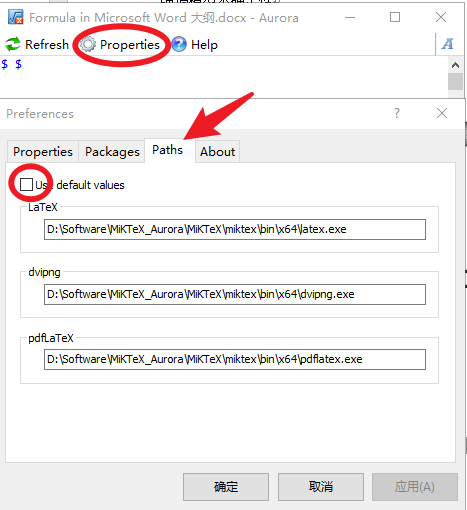这时就可以写一些简单的代码了。比如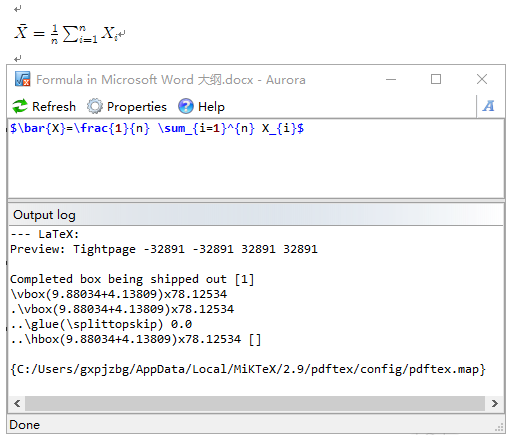3 伪代码配置

伪代码需要安装额外的包，网上常见的说法是在 Packages 中输入，但自己一直报错说

problems running latex

3.1 设置 Rendering method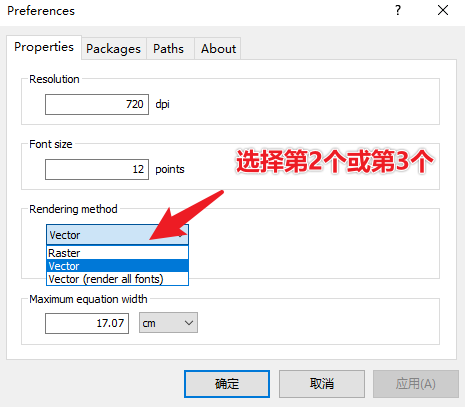3.2 【重要】安装包！！！
自己一开始安装网上教程一直不成功的原因就是这里。
管理员身份运行 miktex-console.exe ，自己的位置在

D:\Software\MiKTeX_Aurora\MiKTeX\miktex\bin\x64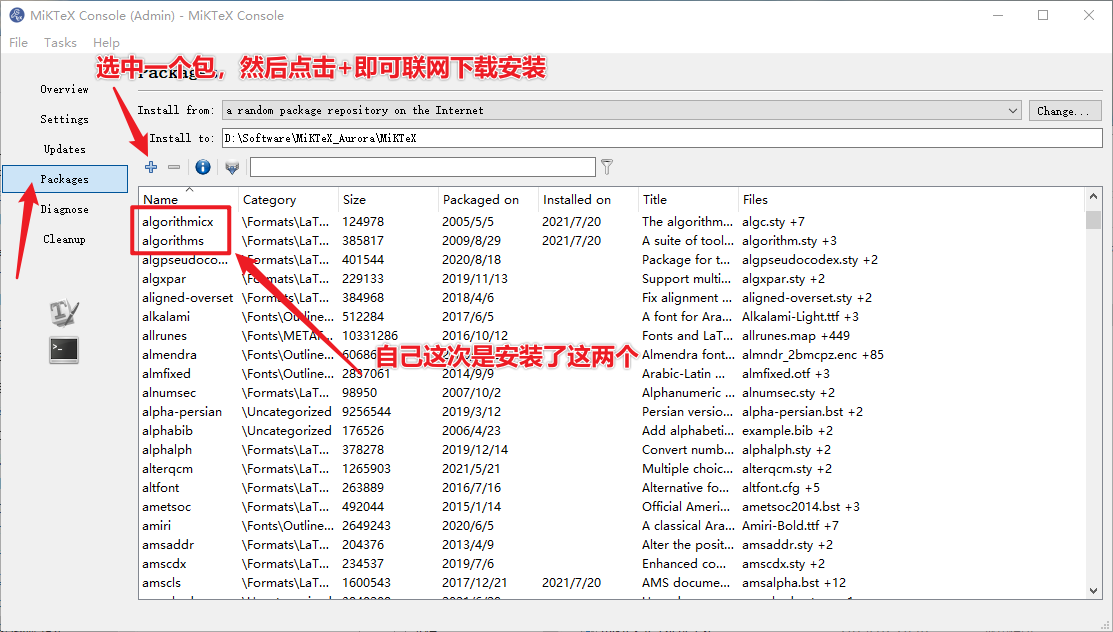3.3 添加包
这个网上有很多示例了，差别不大。下面摘录几个，备用。
自己用的是第二个。第一个的包看上去更多一些，但常见的代码用第二个就够用了。
如果用第一个的话还是会报错 problems running latex，应该是还需要下载别的库，自己就先不折腾了。

\usepackage{amsmath}
\usepackage{amssymb}
% \usepackage{euler}
\providecommand{\abs}{\left\lvert#1\right\rvert}
\providecommand{\norm}{\left\lVert#1\right\rVert}
\usepackage{bbm}
\usepackage{CJK}
\usepackage{listings}
\usepackage{xcolor}
\usepackage{listings}
\usepackage{amsmath,bm,graphicx,multirow,bm,bbm,amssymb,psfrag,algorithm,subfigure,color,mdframed,wasysym,subeqnarray,multicol}

\usepackage{algorithm}
\usepackage{algpseudocode}
\usepackage{amsmath}
\renewcommand{\algorithmicrequire}{\textbf{Input:}}
\renewcommand{\algorithmicensure}{\textbf{Output:}}

\documentclass{article}

\usepackage{multirow}
\usepackage{algorithm}
\usepackage{algpseudocode}
\usepackage{amsmath}
\usepackage{geometry}
\usepackage{algorithmicx}
\usepackage{algpseudocode}

\renewcommand{\algorithmicrequire}{\textbf{Input:}} % Use Input in the format of Algorithm
\renewcommand{\algorithmicensure}{\textbf{Output:}}

4 编写伪代码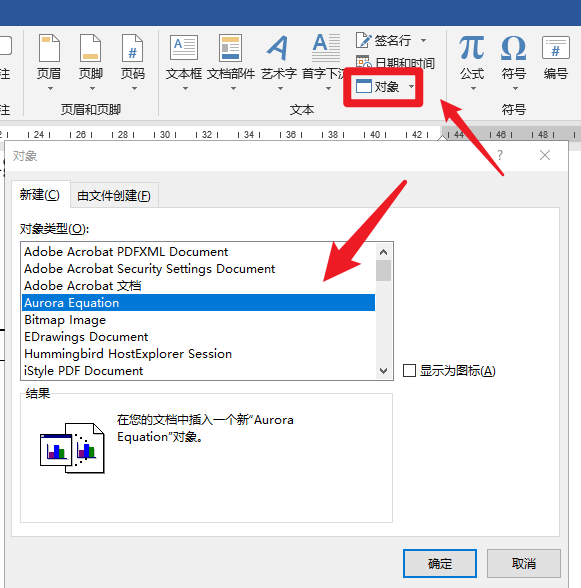下面提供几个示例
示例1

\renewcommand{\thealgorithm}{1}
\begin{algorithm}[H]
\caption{algorithm caption} %算法的名字
\hspace*{0.02in} {\bf Input:} %算法的输入， \hspace*{0.02in}用来控制位置，同时利用 \\ 进行换行
input parameters A, B, C\\
\hspace*{0.02in} {\bf Output:} %算法的结果输出
output result
\begin{algorithmic}
\State some description % \State 后写一般语句
\For{condition} % For 语句，需要和EndFor对应
\State ...
\If{condition} % If 语句，需要和EndIf对应
\State ...
\Else
\State ...
\EndIf
\EndFor
\While{condition} % While语句，需要和EndWhile对应
\State ...
\EndWhile
\State \Return result
\end{algorithmic}
\end{algorithm}

效果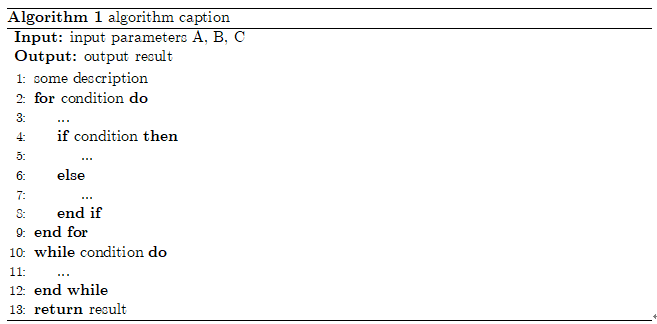示例2

\renewcommand{\thealgorithm}{1}
\begin{algorithm}[H]
\caption{*******************************************}
\label{ABCLFRS}
\begin{algorithmic}
\Require{S,$\lambda$,T,k}
\Ensure{$\mathbf{w}_{222}$}\\
\textbf{initialize}: Set $\mathbf{w}_1 = 0$
\For{$t = 1,2,...,T$}
\State Choose $A_t \subset[m]$
\EndFor
\end{algorithmic}

\end{algorithm}

效果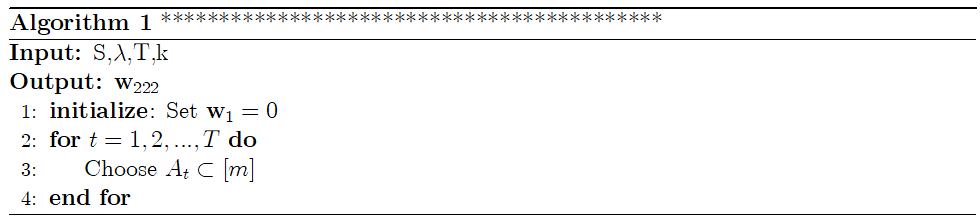示例3

\begin{algorithm}[H]
\caption{algorithm1}
\begin{algorithmic}
\Require
Enter .....;
\Ensure
Outpur......
\State state1......
\State state2......
\State state3......
\While{(a$>$b)}

\State  state4......
\If { c$<$d}
\State state5......
\Else
\State state6......
\EndIf
\State state7......
\EndWhile
\For{aaa}
\State state8......
\EndFor
\end{algorithmic}
\end{algorithm}

效果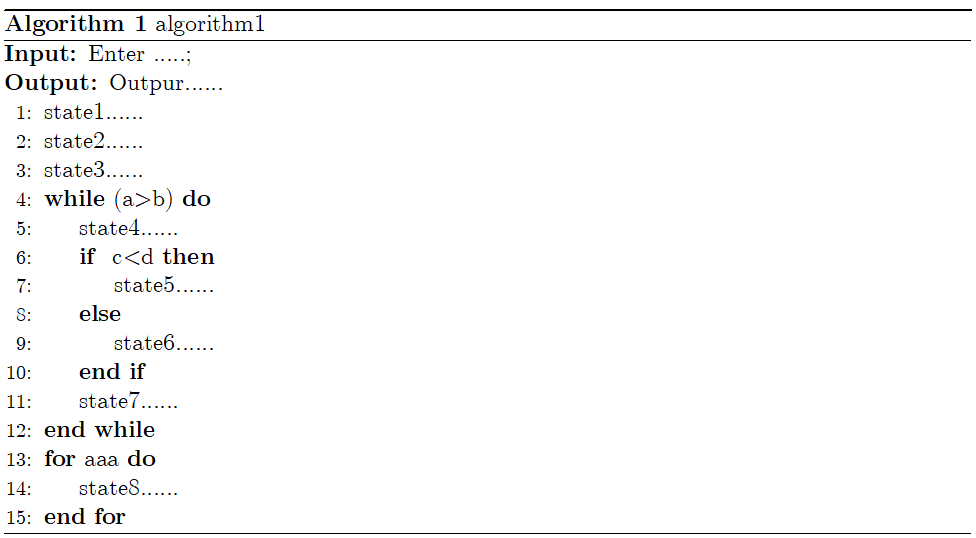参考

展开全文auroralatex 伪代码
• 如何在word中写伪代码 通过下面的链接，可以知道怎样在word中使用aurora 使用Aurora在Word中插入算法伪代码教程！！！亲测有效！！！写论文必备！！！ 一定要注意的一点： 之前看的教程说aurora安装好之后会...word中写伪代码 Aurora Texlive
• word2016中写出伪代码

千次阅读 2020-03-30 19:14:03
• 这里主要是想说一下如何在word中插入算法的伪代码。 一、使用Aurora插件 二、使用表格doc
• 写论文的时候，时常需要我们将算法以伪代码的形式展示出来，我们都知道latex可以帮我们生成一个漂亮的伪代码格式，但是在word中我们该如何加入呢？ 可以使用Aurora插件实现。Latex in word 1. 插入算法伪代码 在...
• 亲测可用，参考链接：https://blog.csdn.net/LIN_SHUQING/article/details/78896623?utm_source=blogxgwz1
• word中安装插件Aurora写伪代码

千次阅读 2019-09-28 16:55:35
Aurora是32位的，所以office需要为32位， 否则没有操作选项 Miklex需要为32位 Aurora注册机
• 因为我们在书写英文论文时，为增加论文的丰富度，一般会选择插入伪代码，那么伪代码怎么插入才好看，首先推荐的还是Latex，但是吧，我想在word里进行排版,所以，可以选择Aurora插件。 现在网络解决Problems running ...Aurora miktex
• word自动编号怎么实现先写带有编号的内容,然后在加一页目录,把你的问题，可用自动生成目录解决.要想自动生成题目，打文件时应设置题目格式。一、输入题目时这样操作 1、打开WORD文档输入题目时，点菜单字体栏左侧...
• Mac：TexStudio 插入伪代码

千次阅读 2017-12-30 17:06:47
\documentclass{article}\usepackage{algorithm} %需要用的库 \usepackage{algpseudocode} \begin{document}\begin{algorithm} \begin{algorithmic} \Procedure{gcf}{$a,$b}\Comment{The gcd function}mac Texstudio 伪代码
• 如果希望在论文中使用 latex 编写伪代码，就像下图一样简洁美观的话，可以考虑继续阅读。 下载安装与配置 Aurora 1. 确保安装好了 latex 前面介绍了安装一个很大的包 latexlive 环境安装与快速上手，这一个步骤...latex
• 如何在word中插入latex公式和伪代码

万次阅读 多人点赞 2017-12-25 20:42:36
如何在word中插入latex公式和伪代码word中可以通过软件Aurora把latex公式直接搬到word里面，超级好用，安装完软件之后，word的工具栏会自动出现该选项。至于伪代码，只要复制，点击工具栏的 Paste from Tex 就可以...latex word
• -> Ctex -> Settings(Admin) -> Refresh FNDB" 3、安装 Inkspace （将生成的 算法步骤图/伪代码.pdf 转成能导入word的格式）https://inkscape.org/release/inkscape-0.92.4/ 好吧，开始操作~~~ 装好 CTEX 后，打开 ...
• WPS插入LaTeX伪代码

万次阅读 2018-05-20 12:03:24
本来一直都是用word写论文，最近需要插入伪代码，网上搜了下可以安装一个插件，叫做 Aurora，具体方法参考百度经验“如何在WPS或word中插入伪代码”但是上文没有给参考程序，于是博主直接找的 LaTeX 写伪代码的程序...
• 前提说明 1、Aurora好像只支持在32位Word中菜单栏显示加载项； 2、所有安装包下载见此处 安装步骤 详情请见：http://www.yuerya.me/post/aurora-|-latex-in-wordWord Aurora
• 回到Word，就会发现插入伪代码成功！！！ 到这里，就结束了，剩下的工作就是学会使用这种语法，将自己的伪代码放到论文中去！ 如果百度网盘链接失效（我设置了永久有效）或者哪里有问题可以留言。
• 不过如果我们想把论文投向日益发展不错的国内期刊，那么问题就来了，国内期刊有些要求论文的Word格式，且给出的也是Word模板，如果我们还想保留Latex帅气的算法怎么办，莫慌，可以按如下要求操作： 1.你需要下载一...latex
• 在word中插入格式漂亮的代码

千次阅读 2020-03-22 18:01:33
最近在工作中，需要在详细设计文档中插入伪代码。尝试编辑了不同的格式，总是觉得代码显示的不美观，因此尝试在网上搜索一些方法，还真有这方面的资料。如下 一、CSDN的博文《如何优雅的在 Microsoft word中插入...
• 【需求】在用word写小论文的时候想插入漂亮的伪代码，搜索发现有latex这个排版软件可以实现。【思路】1、利用Aurora插件在word中插入对象时打开latex编辑。(这个出现“problems running latex”多种方法均未被解决，...
• 1.打开 http://www.planetb.ca/syntax-highlight-word 2.粘贴代码在Code框内，选择语言，再点击生成代码 3.生成代码格式如下。 4.把代码复制粘贴到word中，搞定！ ...
• Word中使用Aurora插件插入latex伪代码下载以及配置word中的Aurora下载Aurora插件下载latex工具配置latex路径使用 下载以及配置word中的Aurora 下载Aurora插件 首先需要下载Aurora插件，下载地址： 链接：...latex...